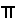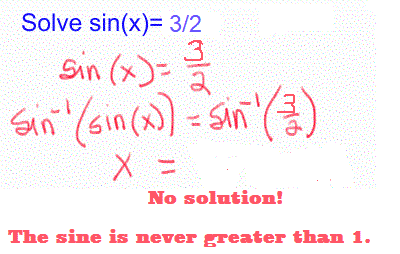## Arc and arc functions

 An arc function undoes a trig or hyperbolic trig function.       Strictly speaking, the symbol sin-1( ) or Arcsin( ) is used for the Arcsine function, the function that undoes the sine. This function returns only one answer for each input and it corresponds to the blue arcsine graph at the left.       Arcsine may be thought of as "the angle whose sine is" making arcsine(1/2) mean "the angle whose sine is 1/2" or/6.       The symbol sin-1( ) is used often when one wishes more than one or even all the values possible even though these values are not covered by the Arcsine function. See below for a better understanding of this.       Think of the Arcsine as the principal arcsine.

 Restrict the domain to take this inverse function.       A function can only be an inverse if it is 1-to-1 and undoes exactly the desired function. See inverse function notes for a review of inverse functions.       In the graph at the left, notice that the sine function, pink and dashed, is not 1-to-1 because it is periodic and repeats every 2.       The only way for f(x) = sin(x) to undo g(x)= sin-1(x) is if it is 1-to-1 which requires the domain to be restricted.       Once this is done f(x) = sin(x) undoes g(x)= sin-1(x) and g(x)= sin-1(x) undoes f(x) = sin(x).       If we only use the pink part, from -to +, then when the restricted sine is reflected over the line y=x to take the inverse graphically, the inverse, g(x) = sin-1(x) is found and will indeed give us a single value for every x value from -1 to 1, inclusive.       This restriction makes the domain of the Arcsine -< x <+and the range to -1< y < 1 as is needed.

 Exactly How Does That Work?The arcsine of a positive number is a first quadrant angle, sin-1(+) is in quadrant I.       The arcsine of zero is zero, sin-1(0) is 0.       The arcsine of a negative number is a negative first quadrant angle, sin-1(-) is in quadrant -I, a clockwise-angle of less than or equal to -/2.       The arccosine of a positive number is a first quadrant angle, cos-1(+) is in quadrant I.       The arccosine of zero is/2, cos-1(0) is/2.       The arccosine of a negative number is a second quadrant angle, cos-1(-) is in quadrant II.       The arctangent of a positive number is a first quadrant angle, tan-1(+) is in quadrant I.       The arctangent of zero is zero, tan-1(0) is 0.       The arctangent of a negative number is a negative first quadrant angle, sin-1(-) is in quadrant -I, a clockwise-angle of less than -/2.

When you simplify an expression, be sure to use the Arcsine.

 Simplify. Answers. 1. arcsin(1/2) arcsin(1/2)=/6, 30°, clearly in the range of the Arcsine function 2. arcsin(-1/2) arcsin(-1/2)= -/6, -30°,clearly in the range of the Arcsine function.     Do not use the fourth quadrant angle 11/6, 330°, even though the sine of 330° is -1/2, because 11/6, is not in the range of the Arcsine function. 3. arcsin(sin(x)) x, one function undoes the other 4. sin(arcsin(x)) x, one function undoes the other 5. arcsin(sin(30°)) 30°, the angle whose sine is the sine of 30° is 30° 6. arcsin(sin(210°)) arcsin(sin(210°))=arcsin(-1/2)= -/6, -30° because the answer must be in the range of the arcsine function

 When you solve an equation, be mindful of the domain of the x in the equation and how many solutions you should be looking for.Though the Arcsine function is used to solve the equation, solutions to the equation may not be in the range of the arcsine function.© 6/2017, 4/2019, A2 mathnstuff.com/math/spoken/here/2class/330/arc.htm